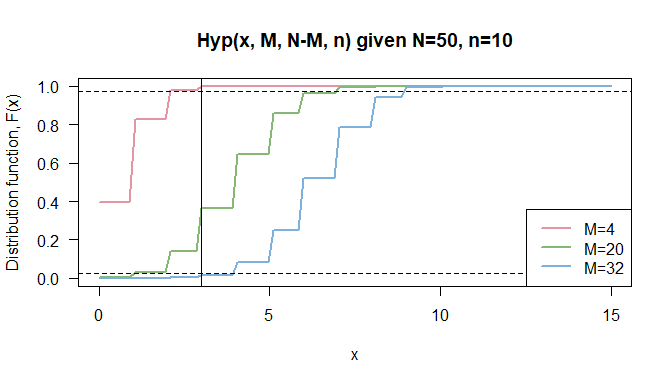# Some Remarks on Sprop

## Exact Hypergeometric Confidence Intervals for Proportion Estimates

In survey sampling on a finite population, a simple random sample is typically selected without replacement, in which case a hypergeometric distribution models the observation. A standard construction for the confidence interval is based on a Normal approximation of the proportion with plug-in estimates for proportion and respective variance.

In most scenarios, this strategy results in satisfactory properties. However, if $$p$$ is close to 0 or 1, it is recommended to use the exact confidence interval based on the hypergeometrical distribution (Kauermann and Kuechenhoff 2010). The Wald-type interval has a coverage probability as low as $$n/N$$ for any $$\alpha$$ (Wang 2015). Therefore, there is no guarantee for the interval to capture the true $$M$$ with the desired confidence level if the sample is much smaller than the population (Wang 2015).

## Implementation in samplingbook

The function samplingbook::Sprop() estimates the proportion out of samples either with or without consideration of finite population correction.

Parameters are

• m an optional non-negative integer for number of positive events,
• n an optional positive integer for sample size,
• N positive integer for population size. Default is N=Inf, which means calculations are carried out without finite population correction.

In case of finite population of size N is provided, different methods for calculating confidence intervals are provided

• approx Wald-type interval based on normal approximation (Agresti and Coull 1998), and
• exact based on hypergeometric distribution as described in more detail in this document.
Sprop(m=3, n = 10, N = 50, level = 0.95)
#>
#> Sprop object: Sample proportion estimate
#> With finite population correction: N = 50
#>
#> Proportion estimate:  0.3
#> Standard error:  0.1366
#>
#> 95% approximate confidence interval:
#>  proportion: [0.0322,0.5678]
#>  number in population: [2,28]
#> 95% exact hypergeometric confidence interval:
#>  proportion: [0.08,0.64]
#>  number in population: [4,32]

## Exact Hypergeometric Confidence Intervals

We observe $$X=m$$, the number of sampled units having the characteristic of interest, where $$X \sim Hyper(M, N, n)$$, with

• $$N$$ is the population size,
• $$M$$ is the number of population units with characteristic of interest, and
• $$n$$ is the given sample size.

The respective density, i.e. the probability of successes in a sample given $$M, N, n$$, is $\Pr(X=m) = \frac{{M \choose m} {N-M \choose n-m}}{N \choose n}, \text{ with support }m \in \{\max(0,n+M-N), \min(M,n)\}$

We want to estimate population proportion $$p = M/N$$, which is equivalent to estimating $$M$$, the total number of population units with some attribute of interest. Then, the boundaries for the exact confidence interval $$[L,U]$$ can be derived as follows:

\begin{aligned} \Pr(X \leq m) & = \sum_{x=0}^m \frac{{U \choose x} {N-U \choose n-x}}{N \choose n} = \alpha_1 \\ \Pr(X \geq m) & = \sum_{x=m}^n \frac{{L \choose x} {N-L \choose n-x}}{N \choose n} = \alpha_2,\\ & \text{with coverage constraint } \alpha_1 + \alpha_2 \leq \alpha \end{aligned} For sake of simplicity, we assume symmetric confidence intervals, i.e $$\alpha_1 = \alpha_2 = \alpha/2$$.

## Some Details on the Implementation

The implementation of the exact confidence interval for proportion estimates uses the hypergeometric distribution function phyper(x, M, N-M, n). Note that the parametrization differs slightly from ours.We search for the optimal confidence boundaries $$[L,U]$$ that fulfill the requirements as defined in the equations above.

• Given known total population $$N$$, sample size $$n$$ and number of successes in the sample $$m$$, we can define some feasibility boundaries for $$M$$:
• Naturally, the smallest possible value is the observed number of successes $$M_{min} = m$$
• The largest possible value equals the total number $$N$$ minus negative observations in the sample, i.e. $$M_{max} = N - (n-m)$$.
• Upper boundary $$U$$
• Start with largest possible value for $$M$$, i.e. $$U_{max} = N - (n-m)$$
• Then, decrease incrementally while the $$\Pr(X \leq m) < \alpha/2$$, so that we find the largest possible value which still fulfills the equation
• Lower boundary $$L$$
• Start with smallest possible value for $$M$$, i.e. $$L_{min} = m$$
• Rewrite $$\Pr(X \geq m) = 1 - \Pr(X \leq m) = \alpha/2 \Leftrightarrow \Pr(X \leq m) = 1 - \alpha/2$$
• Then, increase incrementally while the $$\Pr(X \leq m) \geq 1-\alpha/2$$, so that we find the smallest possible value which still fulfills the equation